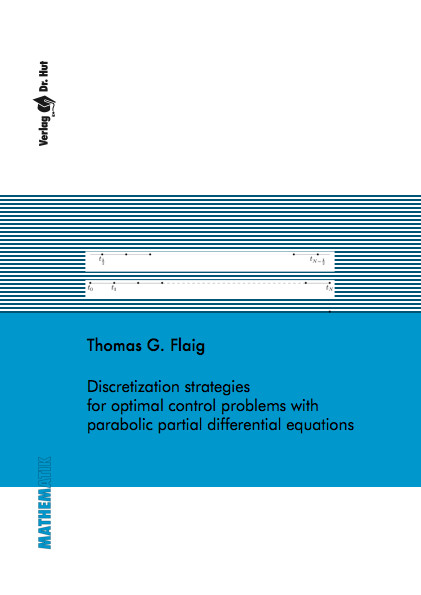Datenbestand vom 25. Mai 2023aktualisiert am 25. Mai 2023

# ISBN 978384391102372,00 € inkl. MwSt, zzgl. Versand

978-3-8439-1102-3, Reihe Mathematik

Thomas G. Flaig
Discretization strategies for optimal control problems with parabolic partial differential equations

158 Seiten, Dissertation Universität der Bundeswehr München (2013), Softcover, A5

## Zusammenfassung / Abstract

The topic of this PhD thesis are estimates for the discretization error of optimal control problems with parabolic partial differential equations. Such problems occur e.g.~during the hydration of young concrete. This problem is introduced in this thesis. Subsequently the discretization of optimal control problems with linear parabolic partial differential equations is discussed in detail.

A focus are tailored Crank-Nicolson schemes with convergence order two. The specialty of these tailored schemes is the commutation of optimization and discretization and the discretization of the state and the control at different discrete times. These discretizations are introduced for abstract parabolic optimal control problems with a cost functional which includes the tracking of a desired state over the full space-time cylinder, the tracking of the terminal state and the control costs.

Furthermore the finite element approximation of some semi-elliptic boundary value problems is discussed. Such problems are connected with parabolic optimal control problems and are of different order of differentiation in different dimensions. The corresponding bilinear form is V-elliptic in an appropriately chosen Hilbert space. For these boundary value problems a regularity estimate and a priori error estimates for the energy norm and L2-norm are proven.

Numerical examples for the Crank-Nicolson discretization and the finite element approximation confirm the expected rates of convergence.